GFG App
Open AppBrowser
Continue

# Clone a Linked List with next and Random Pointer

An example of linked list with a random pointerGiven a linked list of size N where each node has two links: one pointer points to the next node and the second pointer points to any node in the list. The task is to create a clone of this linked list in O(N) time

Note: The pointer pointing to the next node is ‘next‘ pointer and the one pointing to an arbitrary node is called ‘arbit’ pointer as it can point to any arbitrary node in the linked list.

An example of the linked list is shown in the below image: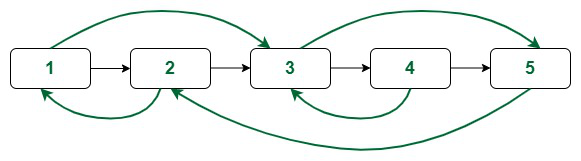An example of linked list with a random pointerAn example of linked list with a random pointer

Approach 1 (Using Extra Space): The idea to solve this problem is:

First create a single linked list with only the ‘next’ pointer and use a mapping to map the new nodes to their corresponding nodes in the given linked list. Now use this mapping to point the arbitrary node from any node in the newly created list.

Follow the steps mentioned beloved to implement the above idea:

• Create a duplicate (say Y) for each node (say X) and map them with corresponding old nodes (say mp, So mp[X] = Y).
• Create the single linked list of the duplicate nodes where each node only has the ‘next’ pointer.
• Now iterate over the old linked list and do the following:
• Find the duplicate node mapped with the current one. (i.e., if the current node is X then duplicate is mp[x])
• Make the arbit pointer of the duplicate node pointing to the duplicate of the current->arbit node (i.e., mp[x]->arbit will point to mp[X->arbit]).
• The linked list created in this way is the required linked list.

Follow the illustration below for a better understanding:

Illustration:

Consider the linked list shown below:The green links are the arbit pointers

Creating copy of Nodes and next pointer:

Initially create single linked list of duplicate nodes with only the next pointers and map them with the old ones.
Here the blue coloured links are used to show the mapping.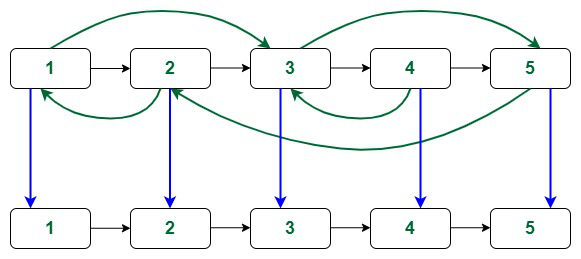New linked list mapped with old nodes

Now iterating the old array and update the arbit pointers as mentioned in the approach. The green coloured links are the arbit pointers.

At first node: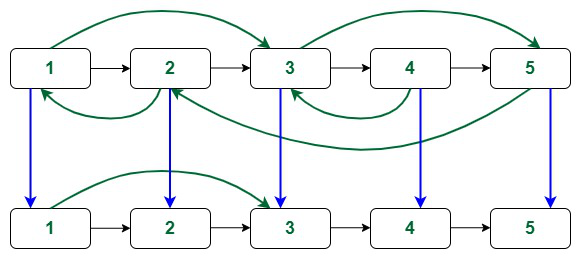Linking arbit pointer of duplicate of 1st node

At second node: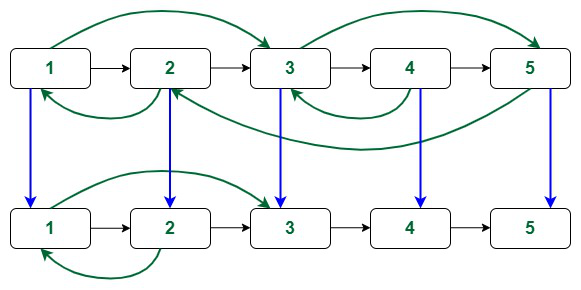Linking arbit pointer of duplicate of 2nd node

At third node: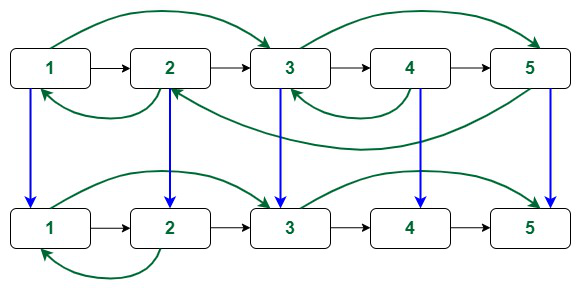Linking arbit pointer of duplicate of 3rd node

At fourth node: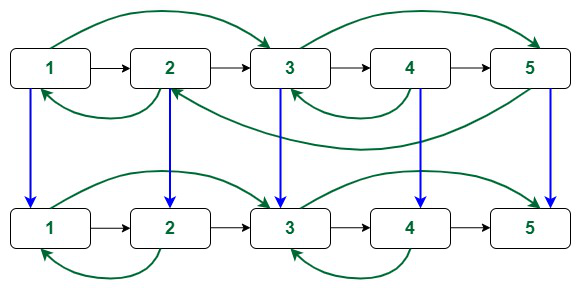Linking arbit pointer of duplicate of 4th node

At fifth node: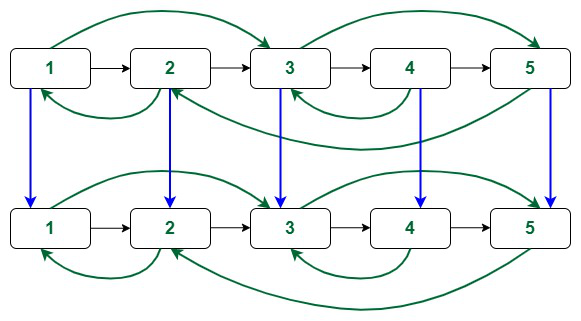Linking arbit pointer of duplicate of 5th node

The final linked list is as shown below: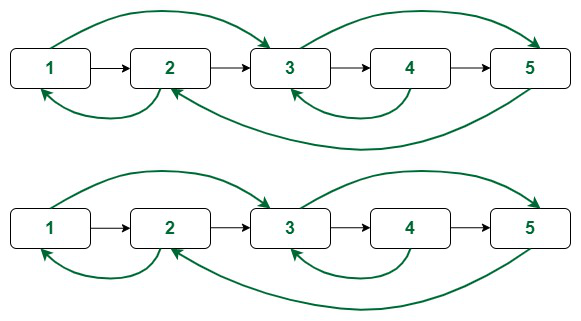The original and the clone

Below is the implementation of the above approach:

## C++14

 `// C++ code to implement the approach`   `#include ` `using` `namespace` `std;`   `// Structure of a node of linked list` `struct` `Node {` `    ``int` `val;` `    ``Node* next;` `    ``Node* arbit;` `  `  `    ``// Constructor` `    ``Node(``int` `x)` `    ``{` `        ``this``->val = x;` `        ``this``->next = NULL;` `        ``this``->arbit = NULL;` `    ``}` `};`   `// Function to clone the linked list` `Node* cloneLinkedList(Node* head)` `{` `    ``// Map to store the mapping of ` `    ``// old nodes with new ones` `    ``unordered_map mp;` `    ``Node *temp, *nhead;` `  `  `    ``// Duplicate of the first node` `    ``temp = head;` `    ``nhead = ``new` `Node(temp->val);` `    ``mp[temp] = nhead;` `  `  `    ``// Loop to create duplicates of nodes ` `    ``// with only next pointer` `    ``while` `(temp->next != NULL) {` `        ``nhead->next ` `            ``= ``new` `Node(temp->next->val);` `        ``temp = temp->next;` `        ``nhead = nhead->next;` `        ``mp[temp] = nhead;` `    ``}` `    ``temp = head;` `  `  `    ``// Loop to clone the arbit pointers` `    ``while` `(temp != NULL) {` `        ``mp[temp]->arbit = mp[temp->arbit];` `        ``temp = temp->next;` `    ``}` `  `  `    ``// Return the head of the clone` `    ``return` `mp[head];` `}`   `// Function to print the linked list` `void` `printList(Node* head)` `{` `    ``cout << head->val << ``"("` `         ``<< head->arbit->val << ``")"``;` `    ``head = head->next;` `    ``while` `(head != NULL) {` `        ``cout << ``" -> "` `<< head->val << ``"("` `             ``<< head->arbit->val << ``")"``;` `        ``head = head->next;` `    ``}` `    ``cout << endl;` `}`   `// Driver code` `int` `main()` `{` `    ``// Creating a linked list with random pointer` `    ``Node* head = ``new` `Node(1);` `    ``head->next = ``new` `Node(2);` `    ``head->next->next = ``new` `Node(3);` `    ``head->next->next->next = ``new` `Node(4);` `    ``head->next->next->next->next ` `        ``= ``new` `Node(5);` `    ``head->arbit = head->next->next;` `    ``head->next->arbit = head;` `    ``head->next->next->arbit ` `        ``= head->next->next->next->next;` `    ``head->next->next->next->arbit ` `        ``= head->next->next;` `    ``head->next->next->next->next->arbit ` `        ``= head->next;` `  `  `    ``// Print the original list` `    ``cout << ``"The original linked list:\n"``;` `    ``printList(head);` `  `  `    ``// Function call` `    ``Node* sol = cloneLinkedList(head);` `  `  `    ``cout << ``"The cloned linked list:\n"``;` `    ``printList(sol);` `  `  `    ``return` `0;` `}`

## Java

 `// Java code to implement the approach`   `import` `java.io.*;` `import` `java.util.HashMap;`   `class` `Node {` `  ``int` `val;` `  ``Node next;` `  ``Node arbit;`   `  ``// Constructor` `  ``Node(``int` `x)` `  ``{` `    ``this``.val = x;` `    ``this``.next = ``null``;` `    ``this``.arbit = ``null``;` `  ``}` `}`   `class` `GFG {`   `  ``static` `Node cloneLinkedList(Node head)` `  ``{` `    ``// Map to store the mapping of` `    ``// old nodes with new ones` `    ``HashMap mp = ``new` `HashMap<>();` `    ``Node temp, nhead;`   `    ``// Duplicate of the first node` `    ``temp = head;` `    ``nhead = ``new` `Node(temp.val);` `    ``mp.put(temp, nhead);`   `    ``// Loop to create duplicates of nodes` `    ``// with only next pointer` `    ``while` `(temp.next != ``null``) {` `      ``nhead.next = ``new` `Node(temp.next.val);` `      ``temp = temp.next;` `      ``nhead = nhead.next;` `      ``mp.put(temp, nhead);` `    ``}` `    ``temp = head;`   `    ``// Loop to clone the arbit pointers` `    ``while` `(temp != ``null``) {` `      ``mp.get(temp).arbit = mp.get(temp.arbit);` `      ``temp = temp.next;` `    ``}`   `    ``// Return the head of the clone` `    ``return` `mp.get(head);` `  ``}`   `  ``static` `void` `printList(Node head)` `  ``{` `    ``System.out.print(head.val + ``"("` `+ head.arbit.val` `                     ``+ ``")"``);` `    ``head = head.next;` `    ``while` `(head != ``null``) {` `      ``System.out.print(``" -> "` `+ head.val + ``"("` `                       ``+ head.arbit.val + ``")"``);` `      ``head = head.next;` `    ``}` `    ``System.out.println();` `  ``}`   `  ``public` `static` `void` `main(String[] args)` `  ``{` `    ``// Creating a linked list with random pointer` `    ``Node head = ``new` `Node(``1``);` `    ``head.next = ``new` `Node(``2``);` `    ``head.next.next = ``new` `Node(``3``);` `    ``head.next.next.next = ``new` `Node(``4``);` `    ``head.next.next.next.next = ``new` `Node(``5``);` `    ``head.arbit = head.next.next;` `    ``head.next.arbit = head;` `    ``head.next.next.arbit = head.next.next.next.next;` `    ``head.next.next.next.arbit = head.next.next;` `    ``head.next.next.next.next.arbit = head.next;`   `    ``// Print the original list` `    ``System.out.println(``"The original linked list:"``);` `    ``printList(head);`   `    ``// Function call` `    ``Node sol = cloneLinkedList(head);`   `    ``System.out.println(``"The cloned linked list:"``);` `    ``printList(sol);` `  ``}` `}`   `/// This code is contributed by lokesh.`

## Python3

 `# Structure of a node of linked list` `class` `Node:` `    ``def` `__init__(``self``, val):` `        ``self``.val ``=` `val` `        ``self``.``next` `=` `None` `        ``self``.arbit ``=` `None`   `# Function to clone the linked list` `def` `cloneLinkedList(head):` `    ``# Map to store the mapping of` `    ``# old nodes with new ones` `    ``mp ``=` `{}` `    ``temp ``=` `head` `    ``nhead ``=` `Node(temp.val)` `    ``mp[temp] ``=` `nhead`   `    ``# Loop to create duplicates of nodes` `    ``# with only next pointer` `    ``while` `temp.``next``:` `        ``nhead.``next` `=` `Node(temp.``next``.val)` `        ``temp ``=` `temp.``next` `        ``nhead ``=` `nhead.``next` `        ``mp[temp] ``=` `nhead`   `    ``temp ``=` `head`   `    ``# Loop to clone the arbit pointers` `    ``while` `temp:` `        ``mp[temp].arbit ``=` `mp[temp.arbit]` `        ``temp ``=` `temp.``next`   `    ``# Return the head of the clone` `    ``return` `mp[head]`   `# Function to print the linked list` `def` `printList(head):` `    ``result ``=` `[]` `    ``while` `head:` `        ``result.append(f``"{head.val}({head.arbit.val})"``)` `        ``head ``=` `head.``next` `    ``print``(``" -> "``.join(result))`   `# Creating a linked list with random pointer` `head ``=` `Node(``1``)` `head.``next` `=` `Node(``2``)` `head.``next``.``next` `=` `Node(``3``)` `head.``next``.``next``.``next` `=` `Node(``4``)` `head.``next``.``next``.``next``.``next` `=` `Node(``5``)` `head.arbit ``=` `head.``next``.``next` `head.``next``.arbit ``=` `head` `head.``next``.``next``.arbit ``=` `head.``next``.``next``.``next``.``next` `head.``next``.``next``.``next``.arbit ``=` `head.``next``.``next` `head.``next``.``next``.``next``.``next``.arbit ``=` `head.``next`   `# Print the original list` `print``(``"The original linked list:"``)` `printList(head)`   `# Function call` `sol ``=` `cloneLinkedList(head)`   `print``(``"The cloned linked list:"``)` `printList(sol)`

## C#

 `// C# code to implement the approach` `using` `System;` `using` `System.Collections.Generic;`   `class` `Node {` `    ``public` `int` `val;` `    ``public` `Node next;` `    ``public` `Node arbit;`   `    ``// Constructor` `    ``public` `Node(``int` `x)` `    ``{` `        ``this``.val = x;` `        ``this``.next = ``null``;` `        ``this``.arbit = ``null``;` `    ``}` `}`   `public` `class` `GFG {`   `    ``static` `Node cloneLinkedList(Node head)` `    ``{` `        ``// Map to store the mapping of` `        ``// old nodes with new ones` `        ``Dictionary mp` `            ``= ``new` `Dictionary();` `        ``Node temp, nhead;`   `        ``// Duplicate of the first node` `        ``temp = head;` `        ``nhead = ``new` `Node(temp.val);` `        ``mp[temp] = nhead;`   `        ``// Loop to create duplicates of nodes` `        ``// with only next pointer` `        ``while` `(temp.next != ``null``) {` `            ``nhead.next = ``new` `Node(temp.next.val);` `            ``temp = temp.next;` `            ``nhead = nhead.next;` `            ``mp[temp] = nhead;` `        ``}` `        ``temp = head;`   `        ``// Loop to clone the arbit pointers` `        ``while` `(temp != ``null``) {` `            ``mp[temp].arbit = mp[temp.arbit];` `            ``temp = temp.next;` `        ``}`   `        ``// Return the head of the clone` `        ``return` `mp[head];` `    ``}`   `    ``static` `void` `printList(Node head)` `    ``{` `        ``Console.Write(head.val + ``"("` `+ head.arbit.val` `                      ``+ ``")"``);` `        ``head = head.next;` `        ``while` `(head != ``null``) {` `            ``Console.Write(``" -> "` `+ head.val + ``"("` `                          ``+ head.arbit.val + ``")"``);` `            ``head = head.next;` `        ``}` `        ``Console.WriteLine();` `    ``}`   `    ``static` `public` `void` `Main()` `    ``{`   `        ``// Code` `        ``// Creating a linked list with random pointer` `        ``Node head = ``new` `Node(1);` `        ``head.next = ``new` `Node(2);` `        ``head.next.next = ``new` `Node(3);` `        ``head.next.next.next = ``new` `Node(4);` `        ``head.next.next.next.next = ``new` `Node(5);` `        ``head.arbit = head.next.next;` `        ``head.next.arbit = head;` `        ``head.next.next.arbit = head.next.next.next.next;` `        ``head.next.next.next.arbit = head.next.next;` `        ``head.next.next.next.next.arbit = head.next;`   `        ``// Print the original list` `        ``Console.WriteLine(``"The original linked list:"``);` `        ``printList(head);`   `        ``// Function call` `        ``Node sol = cloneLinkedList(head);`   `        ``Console.WriteLine(``"The cloned linked list:"``);` `        ``printList(sol);` `    ``}` `}`   `// This code is contributed by lokeshmvs21.`

## Javascript

 `// Javascript code to implement the approach` `class Node {` `  ``constructor(val) {` `    ``this``.val = val;` `    ``this``.next = ``null``;` `    ``this``.arbit = ``null``;` `  ``}` `}`   `const cloneLinkedList = (head) => {`   `  ``// Map to store the mapping of old nodes with new ones` `  ``const mp = ``new` `Map();` `  ``let temp = head;` `  ``let nhead = ``new` `Node(temp.val);` `  ``mp.set(temp, nhead);`   `  ``// Loop to create duplicates of nodes with only next pointer` `  ``while` `(temp.next) {` `    ``nhead.next = ``new` `Node(temp.next.val);` `    ``temp = temp.next;` `    ``nhead = nhead.next;` `    ``mp.set(temp, nhead);` `  ``}` `  ``temp = head;`   `  ``// Loop to clone the arbit pointers` `  ``while` `(temp) {` `    ``mp.get(temp).arbit = mp.get(temp.arbit);` `    ``temp = temp.next;` `  ``}`   `  ``// Return the head of the clone` `  ``return` `mp.get(head);` `}`   `const printList = (head) => {` `  ``let str = `\${head.val}(\${head.arbit.val})`;` `  ``head = head.next;` `  ``while` `(head) {` `    ``str += ` -> \${head.val}(\${head.arbit.val})`;` `    ``head = head.next;` `  ``}` `  ``console.log(str);` `}`   `// Creating a linked list with random pointer` `const head = ``new` `Node(1);` `head.next = ``new` `Node(2);` `head.next.next = ``new` `Node(3);` `head.next.next.next = ``new` `Node(4);` `head.next.next.next.next = ``new` `Node(5);` `head.arbit = head.next.next;` `head.next.arbit = head;` `head.next.next.arbit = head.next.next.next.next;` `head.next.next.next.arbit = head.next.next;` `head.next.next.next.next.arbit = head.next;`   `// Print the original list` `console.log(``"The original linked list:"``);` `printList(head);`   `// Function call` `const sol = cloneLinkedList(head);`   `console.log(``"The cloned linked list:"``);` `printList(sol);`   `// This code is contributed by shivamsharma215`

Output

```The original linked list:
1(3) -> 2(1) -> 3(5) -> 4(3) -> 5(2)
1(3) -> 2(1) -> 3(5) -> 4(3) -> 5(2)```

Time Complexity: O(N)
Auxiliary Space: O(N)

Approach 2 (Using Constant Extra Space):  The idea to solve is using constant extra space is:

Create duplicate of a node and insert it in between that node and the node just next to it.

Now for a node X its duplicate will be X->next and the arbitrary pointer of the duplicate will point to X->arbit->next [as that is the duplicate of X->arbit]

Follow the steps mentioned below to implement the idea:

• Create the copy of node 1 and insert it between node 1 and node 2 in the original Linked List, create the copy of node 2 and insert it between 2nd and 3rd node and so on. Add the copy of N after the Nth node
• Now copy the arbitrary link in this fashion:

original->next->arbitrary = original->arbitrary->next

• Now restore the original and copy linked lists in this fashion in a single loop.

original->next = original->next->next;
copy->next = copy->next->next;

• Make sure that the last element of original->next is NULL.

## C++

 `// C++ code to implement the approach`   `#include ` `using` `namespace` `std;`       `struct` `Node {` `    ``int` `data;` `    ``Node *next, *random;` `    ``Node(``int` `x) {` `        ``data = x;` `        ``next = random = NULL;` `    ``}` `};`   `Node* cloneLinkedList(Node* head) {` `    ``if` `(head == NULL) {` `        ``return` `NULL;` `    ``}` `    `  `    ``// Step 1: Create new nodes and insert them next to the original nodes` `    ``Node* curr = head;` `    ``while` `(curr != NULL) {` `        ``Node* newNode = ``new` `Node(curr->data);` `        ``newNode->next = curr->next;` `        ``curr->next = newNode;` `        ``curr = newNode->next;` `    ``}` `    `  `    ``// Step 2: Set the random pointers of the new nodes` `    ``curr = head;` `    ``while` `(curr != NULL) {` `        ``if` `(curr->random != NULL) {` `            ``curr->next->random = curr->random->next;` `        ``}` `        ``curr = curr->next->next;` `    ``}` `    `  `    ``// Step 3: Separate the new nodes from the original nodes` `    ``curr = head;` `    ``Node* clonedHead = head->next;` `    ``Node* clonedCurr = clonedHead;` `    ``while` `(clonedCurr->next != NULL) {` `        ``curr->next = curr->next->next;` `        ``clonedCurr->next = clonedCurr->next->next;` `        ``curr = curr->next;` `        ``clonedCurr = clonedCurr->next;` `    ``}` `    ``curr->next = NULL;` `    ``clonedCurr->next = NULL;` `    `  `    ``return` `clonedHead;` `}`     `// Function to print the linked list` `void` `printList(Node* head)` `{` `    ``cout << head->data << ``"("` `        ``<< head->random->data << ``")"``;` `    ``head = head->next;` `    ``while` `(head != NULL) {` `        ``cout << ``" -> "` `<< head->data << ``"("` `            ``<< head->random->data << ``")"``;` `        ``head = head->next;` `    ``}` `    ``cout << endl;` `}`   `// Driver code` `int` `main()` `{` `    ``// Creating a linked list with random pointer` `    ``Node* head = ``new` `Node(1);` `    ``head->next = ``new` `Node(2);` `    ``head->next->next = ``new` `Node(3);` `    ``head->next->next->next = ``new` `Node(4);` `    ``head->next->next->next->next` `        ``= ``new` `Node(5);` `    ``head->random = head->next->next;` `    ``head->next->random = head;` `    ``head->next->next->random` `        ``= head->next->next->next->next;` `    ``head->next->next->next->random` `        ``= head->next->next;` `    ``head->next->next->next->next->random` `        ``= head->next;` `    `  `    ``// Print the original list` `    ``cout << ``"The original linked list:\n"``;` `    ``printList(head);` `    `  `    ``// Function call` `    ``Node* sol = cloneLinkedList(head);` `    `  `    ``cout << ``"The cloned linked list:\n"``;` `    ``printList(sol);` `    `  `    ``return` `0;` `}`

## Java

 `class` `Node {` `    ``int` `data;` `    ``Node next, random;`   `    ``Node(``int` `x) {` `        ``data = x;` `        ``next = random = ``null``;` `    ``}` `}`   `public` `class` `Main {` `    ``public` `static` `void` `main(String[] args) {` `        ``// Creating a linked list with random pointer` `        ``Node head = ``new` `Node(``1``);` `        ``head.next = ``new` `Node(``2``);` `        ``head.next.next = ``new` `Node(``3``);` `        ``head.next.next.next = ``new` `Node(``4``);` `        ``head.next.next.next.next = ``new` `Node(``5``);` `        ``head.random = head.next.next;` `        ``head.next.random = head;` `        ``head.next.next.random = head.next.next.next.next;` `        ``head.next.next.next.random = head.next.next;` `        ``head.next.next.next.next.random = head.next;`   `        ``// Print the original list` `        ``System.out.println(``"The original linked list:"``);` `        ``printList(head);`   `        ``// Function call` `        ``Node sol = cloneLinkedList(head);`   `        ``System.out.println(``"The cloned linked list:"``);` `        ``printList(sol);` `    ``}`   `    ``public` `static` `Node cloneLinkedList(Node head) {` `        ``if` `(head == ``null``) {` `            ``return` `null``;` `        ``}`   `        ``// Step 1: Create new nodes and insert them next to the original nodes` `        ``Node curr = head;` `        ``while` `(curr != ``null``) {` `            ``Node newNode = ``new` `Node(curr.data);` `            ``newNode.next = curr.next;` `            ``curr.next = newNode;` `            ``curr = newNode.next;` `        ``}`   `        ``// Step 2: Set the random pointers of the new nodes` `        ``curr = head;` `        ``while` `(curr != ``null``) {` `            ``if` `(curr.random != ``null``) {` `                ``curr.next.random = curr.random.next;` `            ``}` `            ``curr = curr.next.next;` `        ``}`   `        ``// Step 3: Separate the new nodes from the original nodes` `        ``curr = head;` `        ``Node clonedHead = head.next;` `        ``Node clonedCurr = clonedHead;` `        ``while` `(clonedCurr.next != ``null``) {` `            ``curr.next = curr.next.next;` `            ``clonedCurr.next = clonedCurr.next.next;` `            ``curr = curr.next;` `            ``clonedCurr = clonedCurr.next;` `        ``}` `        ``curr.next = ``null``;` `        ``clonedCurr.next = ``null``;`   `        ``return` `clonedHead;` `    ``}`   `    ``// Function to print the linked list` `    ``public` `static` `void` `printList(Node head) {` `        ``System.out.print(head.data + ``"("` `+ head.random.data + ``")"``);` `        ``head = head.next;` `        ``while` `(head != ``null``) {` `            ``System.out.print(``" -> "` `+ head.data + ``"("` `+ head.random.data + ``")"``);` `            ``head = head.next;` `        ``}` `        ``System.out.println();` `    ``}` `}`

## Python3

 `class` `Node:` `    ``def` `__init__(``self``, x):` `        ``self``.data ``=` `x` `        ``self``.``next` `=` `None` `        ``self``.random ``=` `None`     `def` `cloneLinkedList(head):` `    ``if` `head ``=``=` `None``:` `        ``return` `None` `    ``# Step 1: Create new nodes and insert them next to the original nodes` `    ``curr ``=` `head` `    ``while` `curr !``=` `None``:` `        ``newNode ``=` `Node(curr.data)` `        ``newNode.``next` `=` `curr.``next` `        ``curr.``next` `=` `newNode` `        ``curr ``=` `newNode.``next` `    ``# Step 2: Set the random pointers of the new nodes` `    ``curr ``=` `head` `    ``while` `curr !``=` `None``:` `        ``if` `curr.random !``=` `None``:` `            ``curr.``next``.random ``=` `curr.random.``next` `        ``curr ``=` `curr.``next``.``next` `    ``# Step 3: Separate the new nodes from the original nodes` `    ``curr ``=` `head` `    ``clonedHead ``=` `head.``next` `    ``clonedCurr ``=` `clonedHead` `    ``while` `clonedCurr.``next` `!``=` `None``:` `        ``curr.``next` `=` `curr.``next``.``next` `        ``clonedCurr.``next` `=` `clonedCurr.``next``.``next` `        ``curr ``=` `curr.``next` `        ``clonedCurr ``=` `clonedCurr.``next` `    ``curr.``next` `=` `None` `    ``clonedCurr.``next` `=` `None` `    ``return` `clonedHead`   `# Function to print the linked list`     `def` `printList(head):` `    ``print``(head.data, ``"("``, head.random.data, ``")"``, end``=``"")` `    ``head ``=` `head.``next` `    ``while` `head !``=` `None``:` `        ``print``(``"->"``, head.data, ``"("``, head.random.data, ``")"``, end``=``"")` `        ``head ``=` `head.``next` `    ``print``()`     `# Driver code` `if` `__name__ ``=``=` `'__main__'``:` `    ``# Creating a linked list with random pointer` `    ``head ``=` `Node(``1``)` `    ``head.``next` `=` `Node(``2``)` `    ``head.``next``.``next` `=` `Node(``3``)` `    ``head.``next``.``next``.``next` `=` `Node(``4``)` `    ``head.``next``.``next``.``next``.``next` `=` `Node(``5``)` `    ``head.random ``=` `head.``next``.``next` `    ``head.``next``.random ``=` `head` `    ``head.``next``.``next``.random ``=` `head.``next``.``next``.``next``.``next` `    ``head.``next``.``next``.``next``.random ``=` `head.``next``.``next` `    ``head.``next``.``next``.``next``.``next``.random ``=` `head.``next`   `    ``# Print the original list` `    ``print``(``"The original linked list:"``)` `    ``printList(head)`   `    ``# Function call` `    ``sol ``=` `cloneLinkedList(head)` `    ``print``(``"The cloned linked list:"``)` `    ``printList(sol)`

## C#

 `using` `System;`   `public` `class` `Node {` `    ``public` `int` `data;` `    ``public` `Node next, random;`   `    ``public` `Node(``int` `x) {` `        ``data = x;` `        ``next = random = ``null``;` `    ``}` `}`   `public` `class` `GFG {`   `    ``public` `static` `Node CloneLinkedList(Node head) {` `        ``if` `(head == ``null``) {` `            ``return` `null``;` `        ``}`   `        ``// Step 1: Create new nodes and insert them next to the original nodes` `        ``Node curr = head;` `        ``while` `(curr != ``null``) {` `            ``Node newNode = ``new` `Node(curr.data);` `            ``newNode.next = curr.next;` `            ``curr.next = newNode;` `            ``curr = newNode.next;` `        ``}`   `        ``// Step 2: Set the random pointers of the new nodes` `        ``curr = head;` `        ``while` `(curr != ``null``) {` `            ``if` `(curr.random != ``null``) {` `                ``curr.next.random = curr.random.next;` `            ``}` `            ``curr = curr.next.next;` `        ``}`   `        ``// Step 3: Separate the new nodes from the original nodes` `        ``curr = head;` `        ``Node clonedHead = head.next;` `        ``Node clonedCurr = clonedHead;` `        ``while` `(clonedCurr.next != ``null``) {` `            ``curr.next = curr.next.next;` `            ``clonedCurr.next = clonedCurr.next.next;` `            ``curr = curr.next;` `            ``clonedCurr = clonedCurr.next;` `        ``}` `        ``curr.next = ``null``;` `        ``clonedCurr.next = ``null``;`   `        ``return` `clonedHead;` `    ``}`   `    ``// Function to print the linked list` `    ``public` `static` `void` `PrintList(Node head) {` `        ``Console.Write(head.data + ``"("` `+ head.random.data + ``")"``);` `        ``head = head.next;` `        ``while` `(head != ``null``) {` `            ``Console.Write(``" -> "` `+ head.data + ``"("` `+ head.random.data + ``")"``);` `            ``head = head.next;` `        ``}` `        ``Console.WriteLine();` `    ``}` `      ``public` `static` `void` `Main() {` `        ``// Creating a linked list with random pointer` `        ``Node head = ``new` `Node(1);` `        ``head.next = ``new` `Node(2);` `        ``head.next.next = ``new` `Node(3);` `        ``head.next.next.next = ``new` `Node(4);` `        ``head.next.next.next.next = ``new` `Node(5);` `        ``head.random = head.next.next;` `        ``head.next.random = head;` `        ``head.next.next.random = head.next.next.next.next;` `        ``head.next.next.next.random = head.next.next;` `        ``head.next.next.next.next.random = head.next;`   `        ``// Print the original list` `        ``Console.WriteLine(``"The original linked list:"``);` `        ``PrintList(head);`   `        ``// Function call` `        ``Node sol = CloneLinkedList(head);`   `        ``Console.WriteLine(``"The cloned linked list:"``);` `        ``PrintList(sol);` `    ``}`   `}`

## Javascript

 `// JavaScript code to implement the approach`   `class Node {` `  ``constructor(x) {` `    ``this``.data = x;` `    ``this``.next = ``null``;` `    ``this``.random = ``null``;` `  ``}` `}`   `function` `cloneLinkedList(head) {` `  ``if` `(head === ``null``) {` `    ``return` `null``;` `  ``}`   `  ``// Step 1: Create new nodes and insert them next to the original nodes` `  ``let curr = head;` `  ``while` `(curr !== ``null``) {` `    ``const newNode = ``new` `Node(curr.data);` `    ``newNode.next = curr.next;` `    ``curr.next = newNode;` `    ``curr = newNode.next;` `  ``}`   `  ``// Step 2: Set the random pointers of the new nodes` `  ``curr = head;` `  ``while` `(curr !== ``null``) {` `    ``if` `(curr.random !== ``null``) {` `      ``curr.next.random = curr.random.next;` `    ``}` `    ``curr = curr.next.next;` `  ``}`   `  ``// Step 3: Separate the new nodes from the original nodes` `  ``curr = head;` `  ``const clonedHead = head.next;` `  ``let clonedCurr = clonedHead;` `  ``while` `(clonedCurr.next !== ``null``) {` `    ``curr.next = curr.next.next;` `    ``clonedCurr.next = clonedCurr.next.next;` `    ``curr = curr.next;` `    ``clonedCurr = clonedCurr.next;` `  ``}` `  ``curr.next = ``null``;` `  ``clonedCurr.next = ``null``;`   `  ``return` `clonedHead;` `}`   `// Function to print the linked list` `function` `printList(head) {` `  ``process.stdout.write(head.data + ``"("` `+ (head.random.data + ``")"``));` `  ``head = head.next;` `  ``while` `(head !== ``null``) {` `    ``process.stdout.write(``" -> "` `+ head.data + ``"("` `+ (head.random.data + ``")"``));` `    ``head = head.next;` `  ``}` `  ``console.log();` `}`   `// Driver code` `// Creating a linked list with random pointer` `const head = ``new` `Node(1);` `head.next = ``new` `Node(2);` `head.next.next = ``new` `Node(3);` `head.next.next.next = ``new` `Node(4);` `head.next.next.next.next = ``new` `Node(5);` `head.random = head.next.next;` `head.next.random = head;` `head.next.next.random = head.next.next.next.next;` `head.next.next.next.random = head.next.next;` `head.next.next.next.next.random = head.next;`   `// Print the original list` `console.log(``"The original linked list:"``);` `printList(head);`   `// Function call` `const sol = cloneLinkedList(head);`   `console.log(``"The cloned linked list:"``);` `printList(sol);`   `// The code is contributed by Arushi Goel.`

Output

```The original linked list:
1(3) -> 2(1) -> 3(5) -> 4(3) -> 5(2)
1(3) -> 2(1) -> 3(5) -> 4(3) -> 5(2)```

Clone a linked list with next and random pointer in O(1) space
Time Complexity: O(N)
Auxiliary Space: O(1)

#### Approach 3(Using Recursion):

The idea is to copy the list first and then fill the random slots with the corresponding elements in the new list.

First let’s copy the list. It is as easy as creating a new list node for every value. But at the same time we need to fill a dictionary where the key is the old node and the value is the new node.

Now let’s start copying the random pointer. Since we already finished copying the list all the old nodes have a pointer to their new node. So to fill the random slot we just need to follow that pointer indexing in the random.

If you can ignore the random index, then it is really easy to deepcopy with recursion or iteration. Random index can be confusing because you don’t know where it is(It could point to the previous element or lat elements)

One thing you can try here is that you can just store the all mapping information about the original node and new node.
Map mp returns the new_node if you provide the original node.

In the recursion, if you call the cloneLiinkedList() function before assigning the random element, then all mapping information should be stored when they call the mp[head->random]. So you don’t need to worry about it is empty.

The implementation of the above approach is:

## C++

 `// C++ code to implement the approach`   `#include ` `using` `namespace` `std;`   `struct` `Node {` `    ``int` `data;` `    ``Node *next, *random;` `    ``Node(``int` `x)` `    ``{` `        ``data = x;` `        ``next = random = NULL;` `    ``}` `};`   `map mp;` `Node* cloneLinkedList(Node* head)` `{` `    ``// We got to the end of the LinkedList.` `    ``if` `(!head) {` `        ``return` `nullptr;` `    ``}` `    ``// Create the node.` `    ``Node* node = ``new` `Node(head->data);` `    ``mp[head] = node;` `    ``// Get to the end of the LinkedList.` `    ``node->next = cloneLinkedList(head->next);` `    ``// We already mapped all nodes, just pick which one` `    ``// fits.` `    ``node->random = mp[head->random];`   `    ``return` `node;` `}`   `// Function to print the linked list` `void` `printList(Node* head)` `{` `    ``cout << head->data << ``"("` `<< head->random->data << ``")"``;` `    ``head = head->next;` `    ``while` `(head != NULL) {` `        ``cout << ``" -> "` `<< head->data << ``"("` `             ``<< head->random->data << ``")"``;` `        ``head = head->next;` `    ``}` `    ``cout << endl;` `}`   `// Driver code` `int` `main()` `{` `    ``// Creating a linked list with random pointer` `    ``Node* head = ``new` `Node(1);` `    ``head->next = ``new` `Node(2);` `    ``head->next->next = ``new` `Node(3);` `    ``head->next->next->next = ``new` `Node(4);` `    ``head->next->next->next->next = ``new` `Node(5);` `    ``head->random = head->next->next;` `    ``head->next->random = head;` `    ``head->next->next->random = head->next->next->next->next;` `    ``head->next->next->next->random = head->next->next;` `    ``head->next->next->next->next->random = head->next;`   `    ``// Print the original list` `    ``cout << ``"The original linked list:\n"``;` `    ``printList(head);`   `    ``// Function call` `    ``Node* sol = cloneLinkedList(head);`   `    ``cout << ``"The cloned linked list:\n"``;` `    ``printList(sol);`   `    ``return` `0;` `}`

Output

```The original linked list:
1(3) -> 2(1) -> 3(5) -> 4(3) -> 5(2)
1(3) -> 2(1) -> 3(5) -> 4(3) -> 5(2)
```

Time Complexity: O(N)
Auxiliary Space: O(N)

Related article:
Clone a linked list with next and random pointer | Set 2

My Personal Notes arrow_drop_up